# Community trajectory analysis

## 1. Introduction

In this vignette you will learn how to conduct community trajectory analysis (CTA), as implemented in package vegclust.

library(vegclust)
library(RColorBrewer)

## 2. Simple example

In this section we describe how to study the trajectories of three sites that have been surveyed four times each. We use a simple example so that geometric calculations can be followed more easily.

### 2.1 Trajectory data

To specify community dynamics, we need three data items: (a) a set of community states (i.e. coordinates in a space $$\Omega$$), described using a distance matrix $$d$$; (b) a vector specifying the site corresponding to each community state; (c) a vector specifying the survey corresponding to each community state. Let’s first define the vectors that describe the site and the survey of each community state:

#Description of sites and surveys
sites = c(1,1,1,1,2,2,2,2,3,3,3,3)
surveys=c(1,2,3,4,1,2,3,4,1,2,3,4)

We then build a matrix with the coordinates corresponding to the community states of the three sites during each survey. We assume that the community space $$\Omega$$ has 2 dimensions:

#Raw data table
xy<-matrix(0, nrow=12, ncol=2)
xy[2,2]<-1
xy[3,2]<-2
xy[4,2]<-3
xy[5:6,2] <- xy[1:2,2]
xy[7,2]<-1.5
xy[8,2]<-2.0
xy[5:6,1] <- 0.25
xy[7,1]<-0.5
xy[8,1]<-1.0
xy[9:10,1] <- xy[5:6,1]+0.25
xy[11,1] <- 1.0
xy[12,1] <-1.5
xy[9:10,2] <- xy[5:6,2]
xy[11:12,2]<-c(1.25,1.0)
cbind(sites,surveys,xy)
##       sites surveys
##  [1,]     1       1 0.00 0.00
##  [2,]     1       2 0.00 1.00
##  [3,]     1       3 0.00 2.00
##  [4,]     1       4 0.00 3.00
##  [5,]     2       1 0.25 0.00
##  [6,]     2       2 0.25 1.00
##  [7,]     2       3 0.50 1.50
##  [8,]     2       4 1.00 2.00
##  [9,]     3       1 0.50 0.00
## [10,]     3       2 0.50 1.00
## [11,]     3       3 1.00 1.25
## [12,]     3       4 1.50 1.00

The matrix of Euclidean distances $$d$$ between community states in $$\Omega$$ is then:

#Distance matrix
D = dist(xy)
D
##            1         2         3         4         5         6         7
## 2  1.0000000
## 3  2.0000000 1.0000000
## 4  3.0000000 2.0000000 1.0000000
## 5  0.2500000 1.0307764 2.0155644 3.0103986
## 6  1.0307764 0.2500000 1.0307764 2.0155644 1.0000000
## 7  1.5811388 0.7071068 0.7071068 1.5811388 1.5206906 0.5590170
## 8  2.2360680 1.4142136 1.0000000 1.4142136 2.1360009 1.2500000 0.7071068
## 9  0.5000000 1.1180340 2.0615528 3.0413813 0.2500000 1.0307764 1.5000000
## 10 1.1180340 0.5000000 1.1180340 2.0615528 1.0307764 0.2500000 0.5000000
## 11 1.6007811 1.0307764 1.2500000 2.0155644 1.4577380 0.7905694 0.5590170
## 12 1.8027756 1.5000000 1.8027756 2.5000000 1.6007811 1.2500000 1.1180340
##            8         9        10        11
## 2
## 3
## 4
## 5
## 6
## 7
## 8
## 9  2.0615528
## 10 1.1180340 1.0000000
## 11 0.7500000 1.3462912 0.5590170
## 12 1.1180340 1.4142136 1.0000000 0.5590170

### 2.2 Display trajectories

To begin our analysis of the three trajectories we display them in an ordination space, using function trajectoryPCoA. Since $$\Omega$$ has only two dimensions, the Principal Coordinates Analysis (PCoA) on $$d$$ displays the complete space:

trajectoryPCoA(D, sites, surveys, traj.colors = c("black","red", "blue"), lwd = 2)
legend("topleft", col=c("black","red", "blue"),
legend=c("Trajectory 1", "Trajectory 2", "Trajectory 3"), bty="n", lty=1, lwd = 2)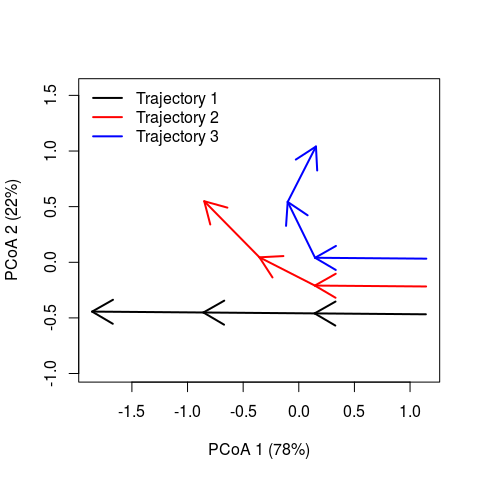While trajectory of site ‘1’ (black arrows) is made of three segments of the same length and direction, trajectory of site ‘2’ (red arrows) has a second and third segments that bend and are shorter than that of the segond segment of site ‘1’. Trajectory of site ‘3’ includes a stronger change in direction and shorter segments.

### 2.3 Trajectory lengths, angles and directionality

We can obtain the length of trajectory segments using function trajectoryLengths and the angles between consecutive segments, using function trajectoryAngles:

trajectoryLengths(D, sites, surveys)
##   S1       S2        S3 Trajectory
## 1  1 1.000000 1.0000000   3.000000
## 2  1 0.559017 0.7071068   2.266124
## 3  1 0.559017 0.5590170   2.118034
trajectoryAngles(D, sites, surveys)
##      S1-S2    S2-S3     mean       sd
## 1 180.0000 180.0000 180.0000 0.000000
## 2 153.4349 161.5651 157.5000 5.748851
## 3 116.5651 126.8699 121.7175 7.286627

where some segments are shorter and angles are smaller for trajectories of site ‘2’ and ‘3’. In this case, the same information could be obtained by inspecting the PCoA representation, but in a case of $$\Omega$$ having many dimensions, the PCoA representation will correspond to a reduced space and hence, angles and lengths will not correspond exactly to those of functions trajectoryLengths and trajectoryAngles, which take into account the full space.

One way of determining the degree to which trajectories follow directional trends (i.e. more or less straight pathways) involves considering all pairs of triplets in the trajectory. This approach is implemented in function trajectoryDirectionality:

trajectoryDirectionality(D, sites, surveys)
##         1         2         3
## 1.0000000 0.9643643 0.7861495

As expected, trajectory of site ‘2’ is less straight than trajectory of site ‘1’ and that of site ‘3’ has the lowest directionality value. Although it would be desirable, the function only computes a descriptive statistic, i.e. it does not perform any statistical test on directionality.

### 2.4 Relative positions within trajectories

Community states occupy a position within their trajectory that depends on their position along the total pathway of the trajectory. By adding the length of segments prior to a given state and dividing the sum by the total length of the trajectory we obtain the relative position of the community state. Function trajectoryProjection allows obtaining the relative position of each point of a trajectory. To use it for this purpose one should use as parameters the distance matrix between state and the indices that conform the trajectory. For example for the two example trajectories we would have:

trajectoryProjection(D, 1:4, 1:4)
##   distanceToTrajectory segment relativePosition
## 1                    0       1        0.0000000
## 2                    0       1        0.3333333
## 3                    0       2        0.6666667
## 4                    0       3        1.0000000
trajectoryProjection(D, 5:8, 5:8)
##   distanceToTrajectory segment relativePosition
## 5                    0       1        0.0000000
## 6                    0       1        0.4412822
## 7                    0       2        0.6879664
## 8                   NA      NA               NA

Because the trajectory of site ‘2’ as the second and third segments that are longer than the first, the relative position of state ‘6’ is lower than 1/3 and that of state ‘7’ is lower than 2/3.

Function trajectoryProjection can also be used to project arbitrary community states on a given trajectory. For example we can study the projection of third state of the trajectory of site ‘1’ (i.e. state 3) onto the trajectory of site ‘2’ (i.e. states 5 to 8):

trajectoryProjection(D, 3, 5:8)
##   distanceToTrajectory segment relativePosition
## 3            0.7071068       3        0.6879664

### 2.5 Trajectory convergence

When trajectories have been sampled the same number of times, function trajectoryConvergence allows performing tests of convergence based on the trend analysis of the sequences of distances between points of the two trajectories (i.e. first-first, second-second, …):

trajectoryConvergence(D, sites, surveys, symmetric = TRUE)
## $tau ## 1 2 3 ## 1 NA 0.9128709 0.9128709 ## 2 0.9128709 NA 0.9128709 ## 3 0.9128709 0.9128709 NA ## ##$p.value
##           1         2         3
## 1        NA 0.1485617 0.1485617
## 2 0.1485617        NA 0.1485617
## 3 0.1485617 0.1485617        NA

Actually, the function performs the Mann-Whitney trend test. Values of the statistic (‘tau’) larger than 0 correspond to trajectories that are diverging, whereas values lower than 0 correspond to trajectories that are converging. By setting symmetric = FALSE the convergence test becomes asymmetric. In this case the sequence of distances is that of points of one trajectory projected onto the other:

trajectoryConvergence(D, sites, surveys, symmetric = FALSE)
## WARNING: Error exit, tauk2. IFAULT =  12
## $tau ## 1 2 3 ## 1 NA 0.9128709 0.9128709 ## 2 0.9128709 NA 0.8164966 ## 3 0.9128709 0.6666666 NA ## ##$p.value
##           1         2         3
## 1        NA 0.1485617 0.1485617
## 2 0.1485617        NA 1.0000000
## 3 0.1485617 0.3081795        NA

### 2.6 Distances between segments and trajectories

To start comparing trajectories between sites, one important step is the calculation of distances between directed segments, which can be obtained by calling function segmentDistances:

Ds = segmentDistances(D, sites, surveys)$Dseg Ds ## 1[1-2] 1[2-3] 1[3-4] 2[1-2] 2[2-3] 2[3-4] ## 1[2-3] 1.0000000 ## 1[3-4] 2.0000000 1.0000000 ## 2[1-2] 0.2500000 1.0307764 2.0155644 ## 2[2-3] 1.0307764 0.7071068 1.5811388 1.0000000 ## 2[3-4] 1.5811388 1.0000000 1.4142136 1.5206906 0.7071068 ## 3[1-2] 0.5000000 1.1180340 2.0615528 0.2500000 1.0307764 1.5000000 ## 3[2-3] 1.1180340 1.1180340 2.0124612 1.0307764 0.5590170 0.7500000 ## 3[3-4] 1.6007811 1.5590170 2.0155644 1.4577380 1.1180340 1.0606602 ## 3[1-2] 3[2-3] ## 1[2-3] ## 1[3-4] ## 2[1-2] ## 2[2-3] ## 2[3-4] ## 3[1-2] ## 3[2-3] 1.0000000 ## 3[3-4] 1.5590170 0.5590170 Distances between segments are affected by differences in both position and direction. Hence, among the six segments of this example, the distance is maximum between the last segment of trajectory ‘1’ and the first segment of trajectory ‘3’. xret = cmdscale(Ds) par(mar=c(4,4,1,1)) plot(xret, xlab="axis 1", ylab = "axis 2", asp=1, pch=21, bg=c(rep("black",3), rep("red",3), rep("blue",3)), xlim=c(-1.5,1), ylim=c(-1,1.5)) text(xret, labels=rep(paste0("s",1:3),3), pos=1) legend("topleft", pt.bg=c("black","red","blue"), pch=21, bty="n", legend=c("Trajectory 1", "Trajectory 2", "Trajectory 3"))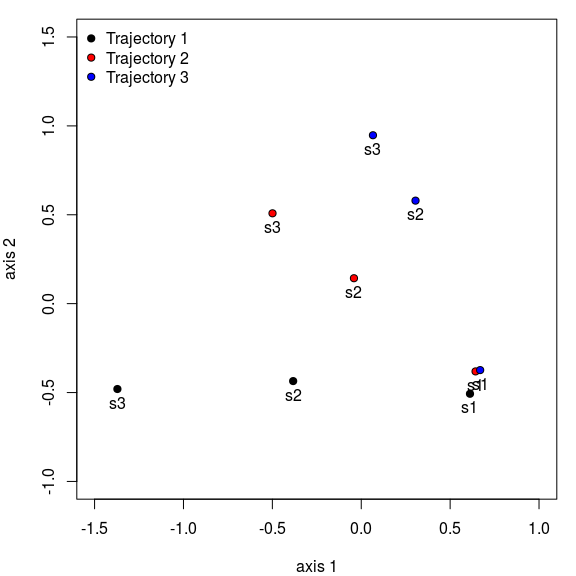Distances between segments are internally calculated when comparing whole trajectories using function trajectoryDistances. Here we show the dissimilarity between the two trajectories as assessed using either the Hausdorff distance (equal to the maximum distance between directed segments) or the directed segment path distance (an average of distances between segments): trajectoryDistances(D, sites, surveys, distance.type = "Hausdorff") ## 1 2 ## 2 2.015564 ## 3 2.061553 1.500000 trajectoryDistances(D, sites, surveys, distance.type = "DSPD") ## 1 2 ## 2 0.7214045 ## 3 1.1345910 0.5714490 ## 3. Structural dynamics in permanent plots In this example we analyze the dynamics of 8 permanent forest plots located on slopes of a valley in the New Zealand Alps. The study area is mountainous and centered on the Craigieburn Range (Southern Alps), South Island, New Zealand. Forests plots are almost monospecific, being the mountain beech (Fuscospora cliffortioides) the main dominant tree species. Previously forests consisted of largely mature stands, but some of them were affected by different disturbances during the sampling period (1972-2009) which includes 9 surveys. We begin our example by loading the data set, which includes 72 plot observations: data("avoca") Community data is in form of an object stratifiedvegdata. To account for differences in tree diameter, while emphasizing regeneration, the data contains individual counts to represent tree abundance and trees are classified in 19 quadratic diameter bins (in cm): {(2.25, 4], (4, 6.25], (6.25, 9], … (110.25, 121]}. The data set also includes vectors avoca_surveys and avoca_sites that indicate the survey and forest plot corresponding to each forest state. Before starting community trajectory analysis, we have to use function vegdiststructto calculate distances between forest plot states in terms of structure and composition: avoca_D_man = vegdiststruct(avoca_strat, method="manhattan", transform = function(x){log(x+1)}) Distances in avoca_D_man are calculated using the Manhattan metric. ### 3.1 Display trajectories in PCoA The distance matrix avoca_D_man conforms our definition of $$\Omega$$. We use trajectoryPCoA to display the relations between forest plot states in this space and to draw the trajectory of each plot: trajectoryPCoA(avoca_D_man, avoca_sites, avoca_surveys, traj.colors = brewer.pal(8,"Accent"), axes=c(1,2), length=0.1, lwd=2) legend("topright", bty="n", legend = 1:8, col = brewer.pal(8,"Accent"), lwd=2)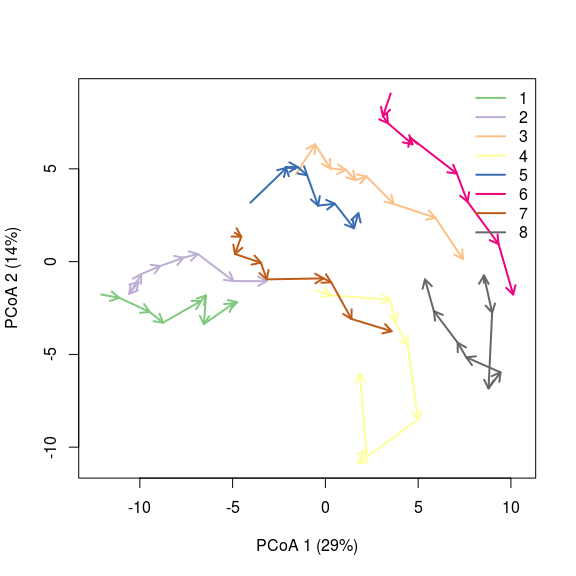Note that in this case, the full $$\Omega$$ include more than two dimensions, and PCoA is representing 43% of total variance (correction for negative eigenvalues is included in the call to cmdscale from trajectoryPCoA), so one has to be careful when interpreting trajectories visually. One can inspect specific trajectories using argument selection in function trajectoryPCoA. This allows getting a better view of particular trajectories, here that of forest plot ‘3’: par(mfrow=c(1,2)) trajectoryPCoA(avoca_D_man, avoca_sites, avoca_surveys, selection= 3, length=0.1, lwd=2) plotTrajDiamDist(3)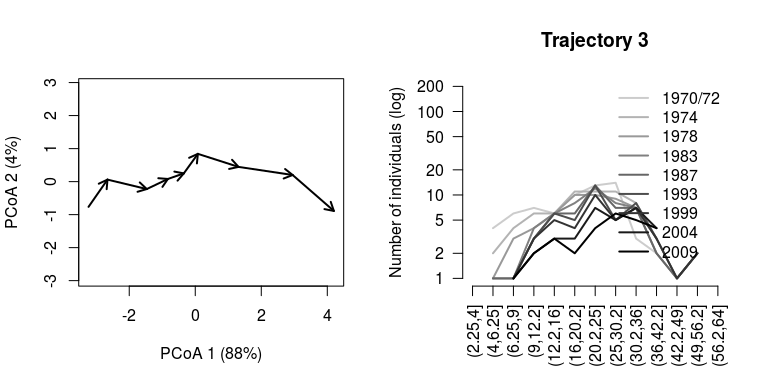In the right hand, we added a representation of the change in the mountain beech tree diameter distribution through time for trajectory of forest plot ‘3’. The dynamics of this plot include mostly growth, which results in individuals moving from one diameter class to the other. The whole trajectory looks mostly directional. Let’s now inspect the trajectory of forest plot ‘4’: par(mfrow=c(1,2)) trajectoryPCoA(avoca_D_man, avoca_sites, avoca_surveys, selection= 4, length=0.1, lwd=2) plotTrajDiamDist(4)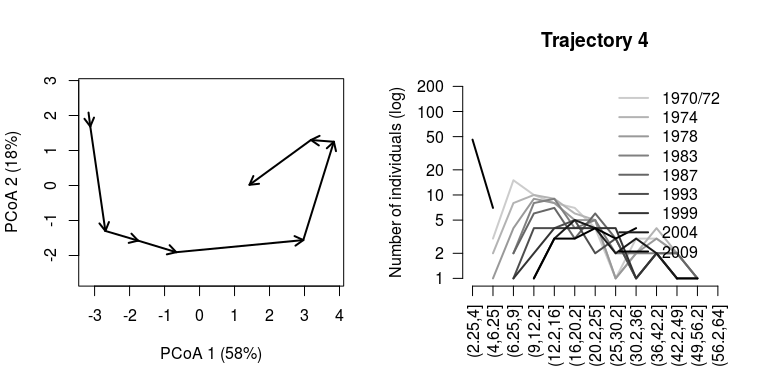This second trajectory is less straight and seems to include a turn by the end of the sampling period, corresponding to the recruitment of new saplings. ### 3.2 Trajectory lengths, angles and directionality While trajectory lengths and angles can be inspected visually in ordination diagrams, it is better to calculate them using the full $$\Omega$$ space (i.e., from matrix avoca_D_man). Using function trajectoryLengths we can see that the trajectory of forest plot ‘4’ is lengthier than that of plot ‘3’, mostly because includes a lengthier last segment (i.e. the recruitment of new individuals): trajectoryLengths(avoca_D_man, avoca_sites, avoca_surveys) ## S1 S2 S3 S4 S5 S6 S7 ## 1 1.2173214 1.5911988 1.0976965 2.1173501 0.5238760 1.5863283 1.5750365 ## 2 0.5971165 1.7469687 0.9866591 0.9413060 1.3687614 0.6280231 1.4565581 ## 3 1.1480971 1.2404953 0.6508116 0.4371405 0.5098385 1.2078811 1.6241741 ## 4 0.7932307 1.8572629 0.7268623 0.8348635 3.0560437 1.9863939 0.9905892 ## 5 1.7769875 0.3367341 0.7283030 0.6515714 1.2660552 0.9544933 1.2605333 ## 6 2.1891568 0.5312711 1.0035212 0.4113220 2.1394743 1.0482871 1.4924056 ## 7 0.2919002 0.8857645 1.0908604 0.5601649 2.0600208 0.3712067 1.1014563 ## 8 0.1909713 1.2000266 2.3211891 0.6539882 2.7807668 0.8170202 1.2072425 ## S8 Trajectory ## 1 0.6277940 10.336602 ## 2 1.1232798 8.848673 ## 3 1.4536390 8.272077 ## 4 3.8794520 14.124698 ## 5 0.5842314 7.558909 ## 6 1.9623777 10.777816 ## 7 1.7518489 8.113223 ## 8 1.5107357 10.681940 If we calculate the angles between consecutive segments (using function trajectoryLengths) we see that indeed the trajectory of ‘3’ is rather directional, but the angles of trajectory of ‘4’ are not that low: trajectoryAngles(avoca_D_man, avoca_sites, avoca_surveys) ## S1-S2 S2-S3 S3-S4 S4-S5 S5-S6 S6-S7 S7-S8 ## 1 1.382519e+02 93.30194 105.98125 153.05862 66.59343 79.32932 77.80037 ## 2 1.118511e+02 145.33494 180.00000 180.00000 154.02889 180.00000 180.00000 ## 3 9.275481e+01 149.11172 180.00000 180.00000 129.09257 131.87146 134.31801 ## 4 1.423426e+02 180.00000 180.00000 143.74760 124.46393 105.78935 130.19668 ## 5 1.379784e+02 108.33600 180.00000 130.04564 114.24103 114.17917 73.87504 ## 6 1.383011e+02 133.88325 123.30359 44.15071 180.00000 180.00000 180.00000 ## 7 1.263675e+02 64.76223 114.80079 119.28558 180.00000 123.74267 180.00000 ## 8 1.909096e-06 180.00000 87.86879 47.63555 108.66052 143.56811 180.00000 ## mean sd ## 1 102.0453 32.57270 ## 2 161.6021 26.30294 ## 3 142.4498 30.79624 ## 4 143.7915 27.76914 ## 5 122.6650 32.41664 ## 6 139.9484 48.82987 ## 7 129.8512 40.11229 ## 8 106.8190 67.49925 By calling function trajectoryDirectionality we can confirm that the trajectory for site ‘4’ is less straight than that of site ‘3’: trajectoryDirectionality(avoca_D_man, avoca_sites, avoca_surveys) ## 1 2 3 4 5 6 7 ## 0.8765513 0.8632656 0.9689678 0.7379840 0.8820482 0.8988080 0.9214587 ## 8 ## 0.7768180 ### 3.3 Distances between trajectories We can calculate the resemblance between forest plot trajectories using trajectoryDistances: ## 1 2 3 4 5 6 7 ## 2 2.405 ## 3 6.805 5.773 ## 4 6.123 6.646 5.225 ## 5 6.020 5.541 3.235 4.966 ## 6 9.490 8.866 3.436 6.043 4.505 ## 7 4.024 3.291 4.365 4.993 4.389 6.205 ## 8 9.797 9.747 5.591 4.611 6.383 5.043 6.949 The closest trajectories are those of plots ‘1’ and ‘2’. They looked rather close in position in the PCoA ordination of $$\Omega$$ with all trajectories, so probably it is position, rather than shape which has influenced this low value. The next pair of similar trajectories are those of the ‘3’-‘5’ pair. We can use cmdscale to produce an ordination of resemblances between trajectories: cmd_D2<-cmdscale(avoca_D_traj_man, add=TRUE, eig=TRUE, k=7) x<-cmd_D2$points[,1]
y<-cmd_D2$points[,2] plot(x,y, type="p", asp=1, xlab=paste0("PCoA 1 (", round(100*cmd_D2$eig/sum(cmd_D2$eig)),"%)"), ylab=paste0("PCoA 2 (", round(100*cmd_D2$eig/sum(cmd_D2\$eig)),"%)"), col="black",
bg= brewer.pal(8,"Accent"), pch=21)
text(x,y, labels=1:8, pos=2)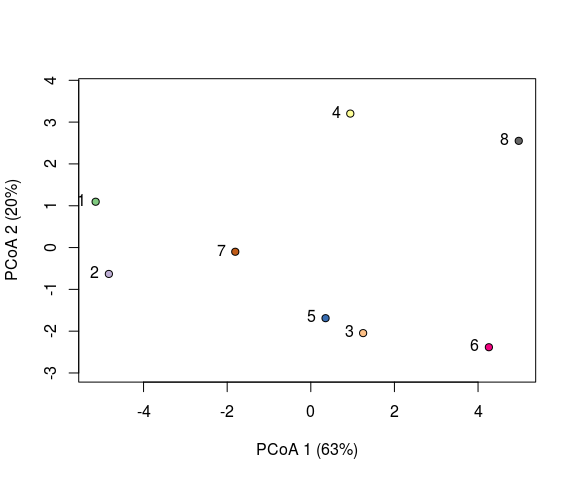## 4. Square root transformation and community trajectories

### 4.1 Introduction

Some dissimilarity coefficients that are popular in community ecology, such as the percentage difference (alias Bray-Curtis), have the drawback of being a non-Euclidean (dissimilarity matrices do not allow a representation in a Cartesian space), or even semi-metric (i.e. triangle inequality is not ensured). In order to use these coefficients in multivariate analyses that require these properties a common transformation is the square root. This document tries to illustrate that applying this transformation has consequences for trajectory analysis.

### 4.2 Simple directional trajectory

Here we use an example of a single synthetic community to illustrate the effect of square root transformation on a community trajectory. We begin by defining the species data of the trajectory itself. The dataset consists of four rows (i.e. surveys) and four columns (species). The dynamics in this example consist in an constant increase in the number of individuals of the first species and a corresponding decrease of the others, while keeping a total abundance of 100 individuals:

sites = rep(1,4)
surveys=1:4
spdata = rbind(c(35,30,20,15),
c(50,25,15,10),
c(65,20,10,5),
c(80,15,5,0))

We now use function vegdist from package vegan to calculate the Bray-Curtis coefficient:

D = vegan::vegdist(spdata, "bray")
D
##      1    2    3
## 2 0.15
## 3 0.30 0.15
## 4 0.45 0.30 0.15

If we draw the resemblance space corresponding to this dissimilarity matrix we see a straight trajectory:

trajectoryPCoA(D,sites,surveys)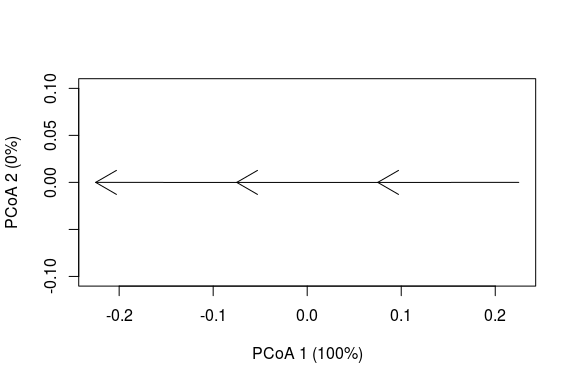Here we see that Bray-Curtis dissimilarity responds linearly to the proposed sequence of community dynamics. To confirm this geometry, we can calculate the geometric properties of the trajectory (i.e. length, angle between consecutive segments and overall directionality):

trajectoryLengths(D,sites,surveys)
##     S1   S2   S3 Trajectory
## 1 0.15 0.15 0.15       0.45
trajectoryAngles(D,sites,surveys)
##   S1-S2 S2-S3 mean sd
## 1   180   180  180  0
trajectoryDirectionality(D,sites,surveys)
## 1
## 1

Angles are 180 degrees and overall directionality is maximum (i.e. 1), in accordance with the plot and the data. We now proceed to take the square root of the dissimilarity values, as would be necessary to achieve a metric (and Euclidean) space in a more complex data set:

sqrtD = sqrt(D)
sqrtD
##           1         2         3
## 2 0.3872983
## 3 0.5477226 0.3872983
## 4 0.6708204 0.5477226 0.3872983

The transformation increases all dissimilarity values (because the original values are smaller than 1), but the increase is larger for smaller values, so the ratio between large dissimilarities and small dissimilarities decreases. This has an effect on the overall shape of the trajectory, which surprisingly now looks like:

trajectoryPCoA(sqrtD,sites,surveys)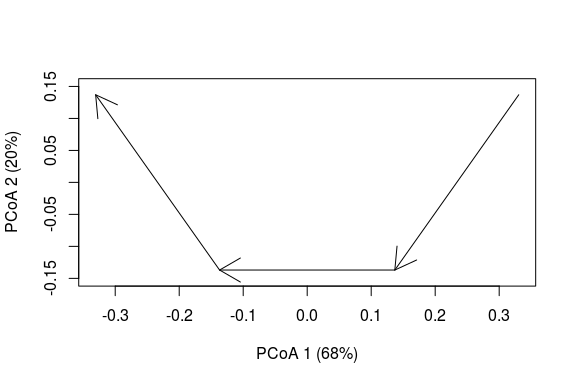In addition to the distortion observed, the number of dimensions of the data have increased (i.e the sum of variance explained by the two axes is 88% < 100%), so we cannot be sure that the angles are well represented. If we re-calculate the properties of the trajectory taking into account all dimensions we obtain:

trajectoryLengths(sqrtD,sites,surveys)
##          S1        S2        S3 Trajectory
## 1 0.3872983 0.3872983 0.3872983   1.161895
trajectoryAngles(sqrtD,sites,surveys)
##   S1-S2 S2-S3 mean sd
## 1    90    90   90  0
trajectoryDirectionality(sqrtD,sites,surveys)
##         1
## 0.7127576

The length of segments and the trajectory have increased, but all segments are still the same size, in agreement with the original trajectory. In contrast, the angles are now 90 degrees and the directionality of the trajectory has decreased substantially.

### 4.3 Longer trajectories

Do the results of the previous section hold for a more complex trajectory? Here we use simulated dynamics to build another trajectory with more species (20) and more time steps. We begin by setting the number of time steps (50) and the size of the community (100 individuals):

Nsteps = 50
CC = 100
Nreplace <- CC*0.05

Nreplace is the number of individuals to be replaced each time step (5%). Now we define the initial community vector and the vector with the probabilities of offspring for each species according to some ecological conditions:

x <- c(0, 1, 0, 67, 1, 3, 0, 2, 2, 2, 1, 6, 2, 0, 0, 2, 5, 1, 6, 0)
poffspring <- c(0, 0, 0.002, 0.661 ,0 ,0, 0.037, 0.281, 0, 0, 0, 0.008, 0, 0, 0.005, 0.003, 0, 0, 0, 0)

We can now simulate the dynamics by sequentially applying stochastic deaths and recruitment:

m <- matrix(0, nrow=Nsteps+1, ncol=length(x))
m[1, ] = x
for(k in 1:Nsteps) {
pdeath <-x/sum(x) #Equal probability of dying
deaths<-rmultinom(1,Nreplace, pdeath)
x <- pmax(x - deaths,0)
offspring = rmultinom(1,Nreplace, as.vector(poffspring))
x <- x + offspring
m[k+1, ]<-x
}

Then we decide how frequently (with respect to the simulated step) a sample of the community is taken, here every four steps:

Sj <- seq(1,51, by=4) #Sample every four steps
mj <- m[Sj,]
surveys = 1:length(Sj)
sites = rep(1,length(Sj))

Now we are ready to calculate the Bray-Curtis dissimilarity and to plot the resulting trajectory:

D <- vegan::vegdist(mj,"bray")
trajectoryPCoA(D, sites, surveys, selection=1,length=0.1, axes=c(1,2))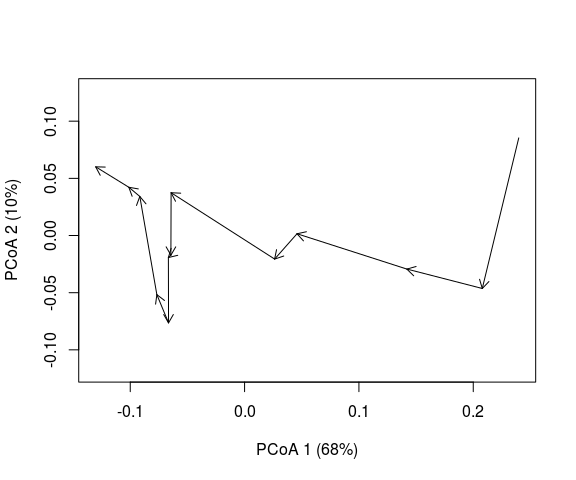The trajectory looks rather directional, although it has some twists derived from stochasticity in death and recruitment. Let’s now look at the square root of the Bray-Curtis dissimilarity:

sqrtD = sqrt(D)
trajectoryPCoA(sqrtD, sites, surveys, selection=1,length=0.1, axes=c(1,2))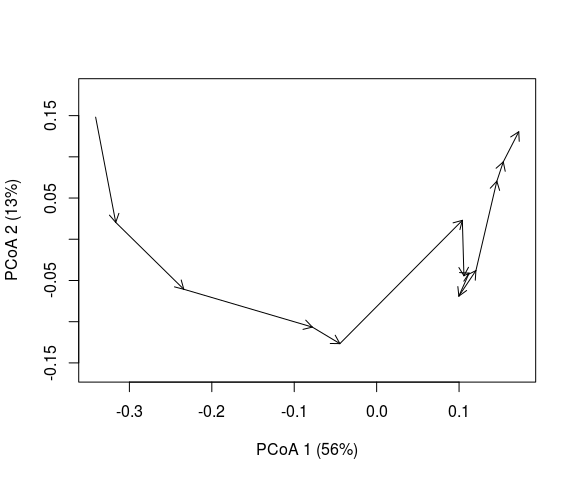While they look different, the differences are not so striking as with the simple example. In other words, the overall shape of the trajectory looks similar in the two plots. From this, we might conclude that the square root transformation did not distort the trajectory significantly. However, note that the proportion of variance explained by the two axes has decreased after the transformation, so there might be changes in trajectory shape that are hidden in the dimensions of the data that we do not see. If we calculate geometric properties we are not limited by the ordination plot and we can take into account all dimensions. In contrast with the ordination plot, the angles of the trajectory are strongly affected by the square root transformation:

trajectoryAngles(D,sites,surveys)
##      S1-S2 S2-S3    S3-S4    S4-S5    S5-S6    S6-S7 S7-S8    S8-S9 S9-S10
## 1 115.3769   180 99.59407 99.59407 61.02847 82.81924   180 46.56746    180
##   S10-S11 S11-S12     mean       sd
## 1     180     180 127.7255 53.33859
trajectoryAngles(sqrtD,sites,surveys)
##     S1-S2 S2-S3    S3-S4    S4-S5    S5-S6    S6-S7 S7-S8    S8-S9 S9-S10
## 1 81.1236    90 78.22177 78.22177 63.77216 75.52249    90 57.97224     90
##   S10-S11 S11-S12     mean       sd
## 1      90      90 80.43946 11.28749

And so does the overall directionality:

trajectoryDirectionality(D,sites,surveys)
##         1
## 0.8875624
trajectoryDirectionality(sqrtD,sites,surveys)
##         1
## 0.6829945

### 4.4 Conclusions

In this small study we have shown how the square root transformation distorts the angles and overall directionality of trajectories on the space defined by the percentage difference (alias Bray-Curtis) dissimilarity index. We suspect that this negative effect of square root transformation on angles happens no matter which coefficient is used to derive the initial distance matrix. Therefore, we advocate for avoiding its use when conducting community trajectory analysis (in particular for angles). However, the square root transformation may still be advised for the representation of (especially complex) trajectories in ordination diagrams, because the alternative is to discard the information corresponding to negative eigenvalues of the distance matrix.